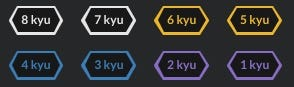CodeWars 是我在走跳的刷題網站，相關介紹請參考 『透過 codewars 修練 Swift 解題技巧』 by 彼得潘的 iOS App Neverland

Codewars 上的題目根據不同程度的定義和要求，分為最簡單的 8 kyu 到最難的 1 kyu，送交自己的答案之後就可以看到其他 Code Warriors 的解法和各種奇思妙想。題目分為最簡單的 8 kyu 到最難的 1 kyu

## 今天來談談這讓我徹夜難眠的一題：難度 6 kyu 的

『深深感受到我和世界的差距』

Spoiler alert! Do not read down if you want to solve this kata by yourself.

Prime 代表質數的意思，除了 1 以外不能被任何數整除，以此延伸來看題目的 “Prime String” 就很容易理解題意。

### JavaScript

``````console.log(primeString("asdf")); // should be true
console.log(primeString("abac")); // should be true
console.log(primeString("qiuefgqiuefgqiuefg")); // should be false
console.log(primeString("zkvjhuj")); // should be true
``````

### 我的方法

``````"qiuefgqiuefgqiuefg".split("q"); // [ '', 'iuefg', 'iuefg', 'iuefg' ]
``````

``````function primeString(s) {
// 將字串重複的部份切成一個規律的陣列
let spArr = s.split(s);
// 切掉不要的首個空元素
spArr.shift();

// 宣告 不重複計數器
let falseCount = 0;

//  將各個元素和它們出現的次數存成 key-value pair
let tCounter = {};
spArr.forEach(x => {
tCounter[x] = (tCounter[x] || 0) + 1;
});

// 將每個元素的重複出現次數清點過後比較，如果出現的次數相同表示有重複
for (let e of spArr) {
// 如果重複次數小於等於 1 表示開頭字母只出現一次，其餘都不重複 falseCount + 1
// 或者有任何元素的出現次數和陣列第一個元素不同 falseCount + 1
tCounter[spArr] <= 1 || tCounter[spArr] !== tCounter[e]
? (falseCount += 1)
: e;
}
// 將不重複計數器 falseCount 轉為布林值輸出，大於 0 就會轉成 true
return !!falseCount;
}
``````

## Best practice & The most Clever

### JavaScript

``````function primeString(s) {
return (s + s).indexOf(s, 1) == s.length;
}
``````

### Ruby

``````def primeString(s)
(s + s).index(s, 1) == s.size
end
``````

steven
012345

stevensteven
01234567891011
~~~~steven

xyxyxyxy
01234567
xyxy--

## Regular expression

### JavaScript

``````function primeString(s) {
return !/^(.+)\1+\$/.test(s);
}
``````

### Ruby

``````def prime_string(s)
s !~ /^(.+)(\1+)\$/
end
``````

## Recursive

Tai An Su 老師提出的遞迴解法，這邊我盡可能地將自己的理解寫在備註中。

### JavaScript

``````function primeString(s, count = 1) {
// 如果字串中有重複的模式，最少也會重複 1 次，故迭代超過字串長度的一半還沒找到就不用找了
if (count > s.length / 2) {
return true;
}

// 每次迭代將字串的一小塊，設定為分割陣列的符號 chunk
let chunk = s.slice(0, count);
// 被 chunk 切過的部分會變成陣列中的空元素，此行判斷是不是能夠將陣列切成重複的小塊
// 如果切完後全部的陣列元素都是空的，代表字串被 chunk 整除，恭喜找到規律。
let grouped = s.split(chunk).every(i => i === "");

// 若上一行的結果為否表示沒有找到重複的規律，則呼叫自己，用更大的單位(count + 1)繼續切，
// 直到超過字串長度的一半都沒找到，代表輸入為 prime string
return grouped ? false : primeString(s, count + 1);
}
``````